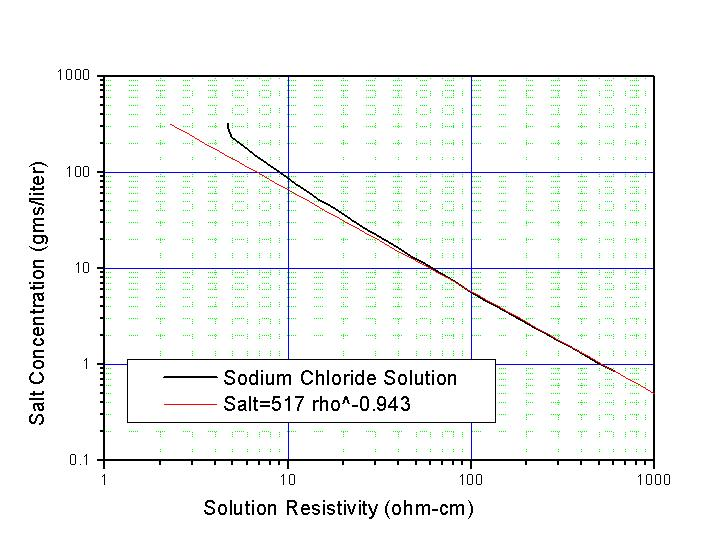# Liquid Resistors

Copper Sulfate
Ammonium Chloride
Sodium Chloride
Sodium Thiosulfate
Liquid resistors are often used in high voltage ac and pulsed power applications. The advantage of these types of resistors is that they can be assembled relatively inexpensively and can absorb large amounts of energy. The disadvantage is that it is somewhat difficult to obtain precise resistance values (rarely better than +/-10%) and the temperature of the solution can vary the resistance significantly. As a result, these resistors are often used in charging systems, dump resistors, etc. in large energy storage capacitor banks where precise resistor values are not necessarily required.

Liquid resistors are usually constructed from tubes of flexible insulating material (such as Tygon) with flat shaped electrodes inserted into both ends and the tube filled with electrolyte solution. Hose clamps or tie wraps seal the tube against the electrode. Electrode material selection depends upon the chosen solution to ensure that contamination of the liquid does not occur over time. A variety of chemical solutions have been used in the past for liquid resistors and four of the most common are shown below in the figure.

Copper Sulfate (CuSO4) Liquid Resistors

Copper sulfate (CuSO4) is often used for liquid resistors. A saturated solution of copper sulfate has a resistivity of ~22 ohm-cm. With this solution, the electrode material should be copper. The graph below shows the salt concentration required for a given copper sulfate solution resistivity (rho) and a curve fitting function to solve for the salt concentration (salt).Copper Sulphate Solution Electrical Resistivity as a Function of Salt Concentration

Ammonium Chloride (NH4Cl) Liquid Resistors

Although copper sulfate solutions are probably the most commonly used liquid resistor, other solutions can also be used depending upon the application requirements. From the figure above, one
can see that the resistivity of ammonium chloride (NH4Cl) solution is lower than the other examples. As a result, this solution is often used for low resistance values. When saturated, the resistivity of this solution is ~2 ohm-cm. Stainless steel (SS304) electrodes are used with this solution. The graph below shows the amount of salt concentration required for a given solution resistivity and a curve fitting equation similar to that above.Ammonium Chloride Solution Electrical Resistivity as a Function of Salt Concentration

Sodium Chloride (NaCl) Liquid Resistors

Sodium chloride (NaCl or table salt) solutions can also be used to make low resistance values. The saturated resistivity of this solution is ~5 ohm-cm and the graph below shows the salt concentration vs. solution resistivity along with a curve fitting function.Sodium Chloride Solution Electrical Resistivity as a Function of Salt Concentration